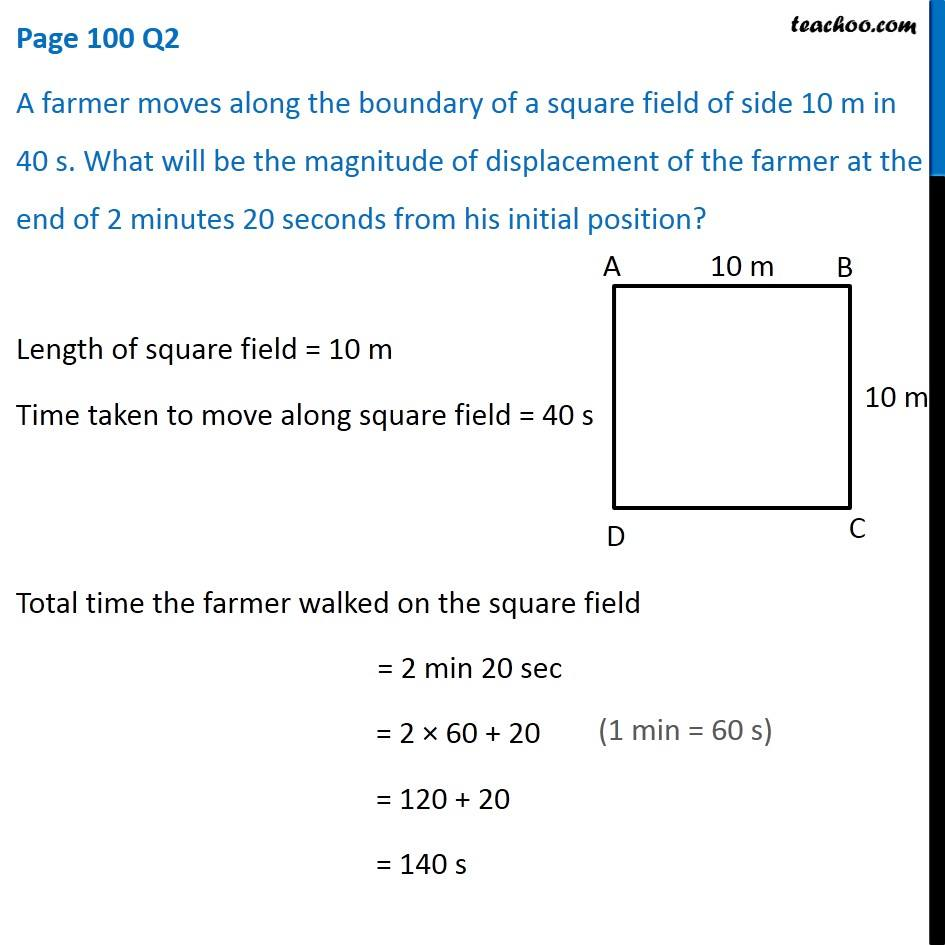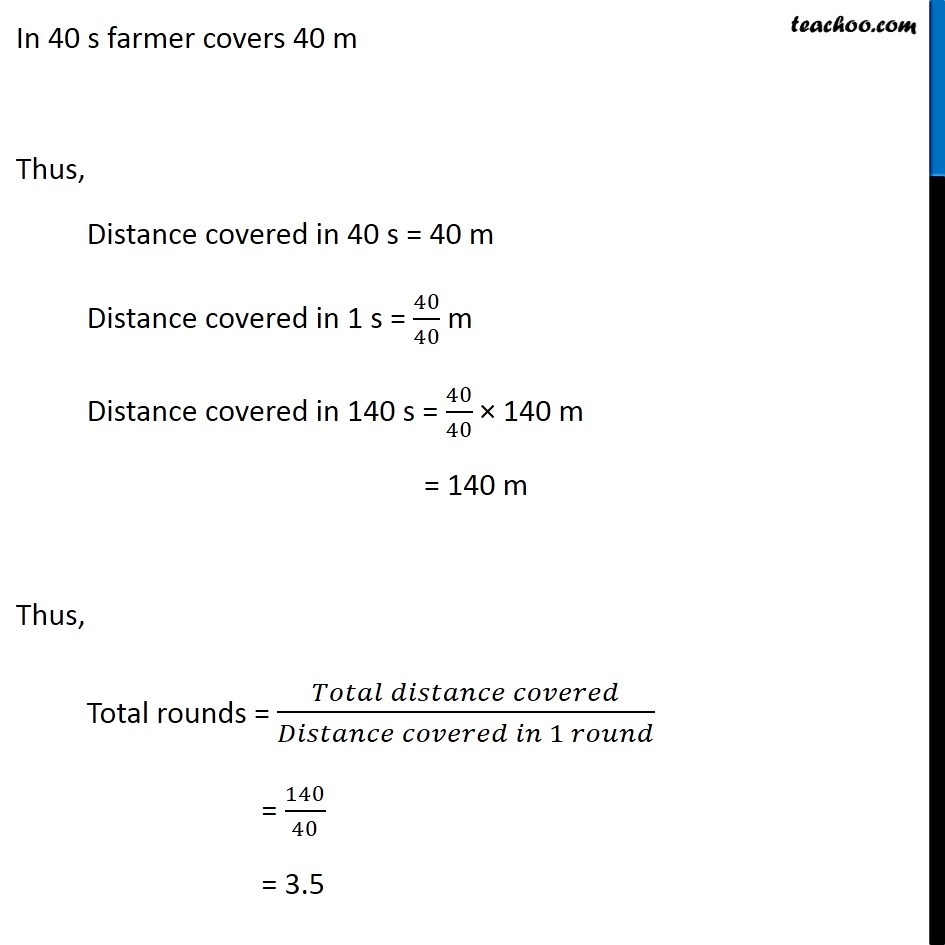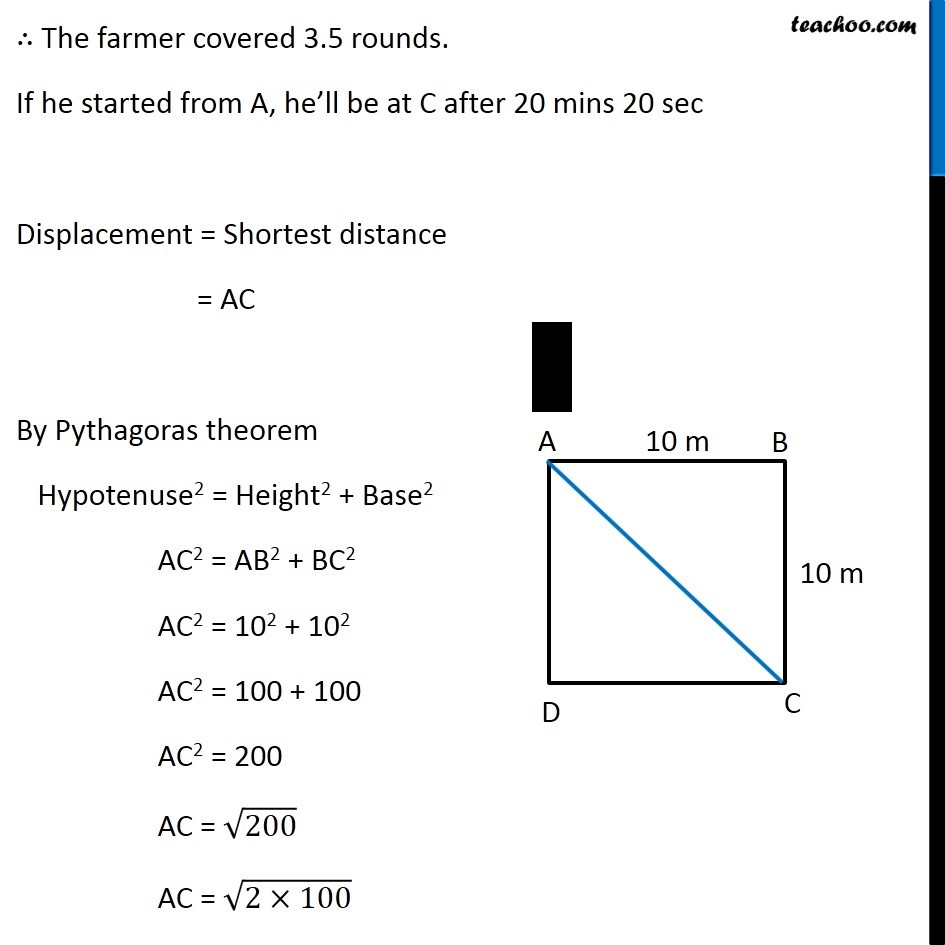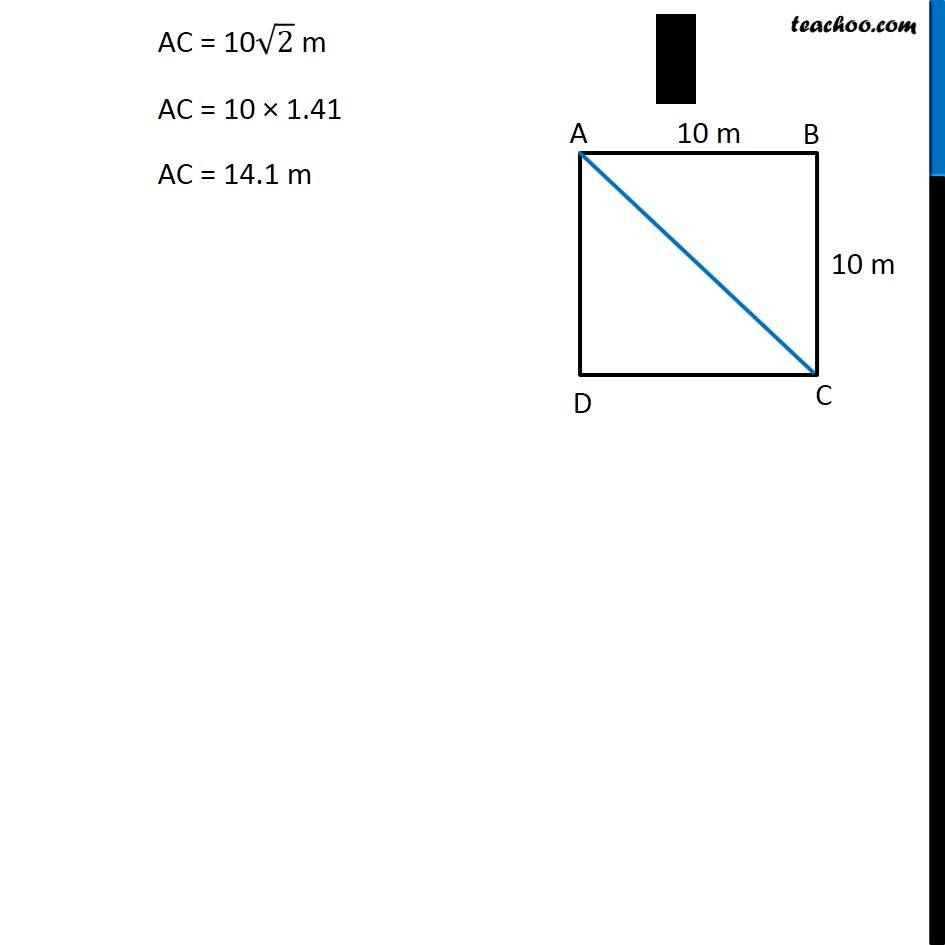Questions from Inside the chapter

Class 9
Chapter 8 Class 9 - MotionLearn in your speed, with individual attention - Teachoo Maths 1-on-1 Class

### Transcript

Page 100 Q2 A farmer moves along the boundary of a square field of side 10 m in 40 s. What will be the magnitude of displacement of the farmer at the end of 2 minutes 20 seconds from his initial position? Length of square field = 10 m Time taken to move along square field = 40 s Total time the farmer walked on the square field = 2 min 20 sec = 2 × 60 + 20 = 120 + 20 = 140 s (1 min = 60 s) In 40 s farmer covers 40 m Thus, Distance covered in 40 s = 40 m Distance covered in 1 s = 40/40 m Distance covered in 140 s = 40/40 × 140 m = 140 m Thus, Total rounds = (𝑇𝑜𝑡𝑎𝑙 𝑑𝑖𝑠𝑡𝑎𝑛𝑐𝑒 𝑐𝑜𝑣𝑒𝑟𝑒𝑑)/(𝐷𝑖𝑠𝑡𝑎𝑛𝑐𝑒 𝑐𝑜𝑣𝑒𝑟𝑒𝑑 𝑖𝑛 1 𝑟𝑜𝑢𝑛𝑑) = 140/40 = 3.5 ∴ The farmer covered 3.5 rounds. If he started from A, he’ll be at C after 20 mins 20 sec Displacement = Shortest distance = AC By Pythagoras theorem Hypotenuse2 = Height2 + Base2 AC2 = AB2 + BC2 AC2 = 102 + 102 AC2 = 100 + 100 AC2 = 200 AC = √200 AC = √(2×100) AC = 10√2 m AC = 10 × 1.41 AC = 14.1 m